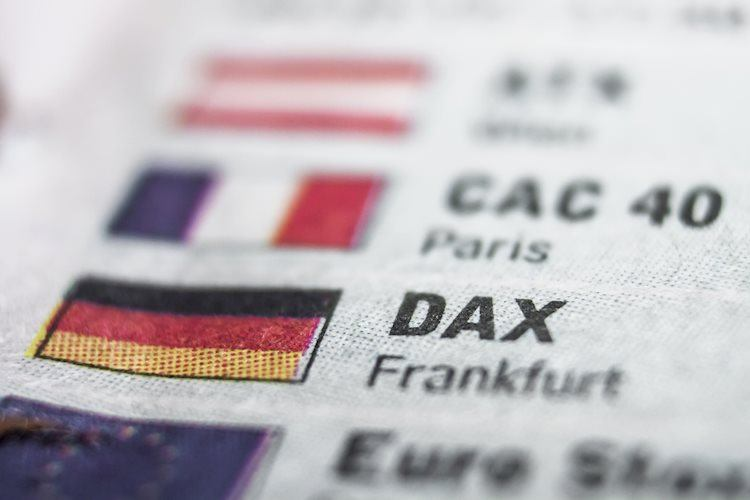# DAX doing three wave corrective bounce from the lows [Video]Share:

The short-term Elliott wave view in the DAX suggests that the index has ended the cycle from the 31 July 2023 peak as a leading diagonal structure in a higher degree corrective sequence. Whereas the decline to 15468 low ended wave 1, wave 2 ended at 16042 high. Wave 3 ended at 14948 low & wave 4 ended at 15575 high. Down from there, wave 5 unfolded in a lesser degree 5 waves structure where wave ((i)) ended at 15103 low. Wave ((ii)) ended at 15288 high, wave ((iii)) ended at 14820 low, wave ((iv)) ended at 14914 high and wave ((v)) ended at 14630 low. Thus ended wave 5 of (A) as a leading diagonal structure.

## DAX 1-hour Elliott Wave chart from 11.03.2023

Up from there, the index is doing a 3-wave corrective bounce within the wave (B) bounce as an Elliott wave zigzag correction. While the initial rally to 14916 high has ended wave ((i)). Then a pullback in wave ((ii)) unfolded as a flat correction where lesser degree wave (a) ended at 14809 low. Wave (b) ended at 14933 high, and wave (c) ended at 14655 low. Above from there, the index is extending higher in wave ((iii)) as an impulse sequence. Near-term, as long as dips remain above 14630 low the index is expected to continue to extend higher for a few more highs. To complete the first leg of the bounce in wave A. Afterward, the index is expected to see a pullback in wave B. Then it should do another extension higher in wave C to complete the 3-wave corrective bounce.